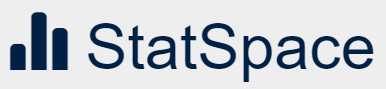# Resources for Teaching and Learning Statistics: Elementary and Secondary Schools

The Canadian branch of an international classroom project to engage students in statistical reasoning, using data collected about themselves and about students from around the world.Information about careers in statistics and resources for teaching statistics from the American Statistical Association.

Online resources promoting statistical literacy across the world, to youth and adults.

Magazine about statistics and how it is usedMagazine about statistics and how it is usedA UK website whose aim is to help improve the way that uncertainty and risk are discussed in society.Blogs on applications of statistics that are bettering our lives, information about careers in statistics, and information on statistically related events and activities worldwide from a global network of nearly 2,360 organizations.Modular resources for use in teaching and learning statistic.

Learning activities developed by Paul Fields

(These activities were presented at the SSC Teacher Workshop in May 2010.)

Compare experimental results with theoretical probability, compare results of probability experiments, display data, collect and interpret data, use graphical representations and numerical summaries to answer questions and interpret data.

Compare experimental results with theoretical probability, compare results of probability experiments, display data, propose and justify inferences and predictions based on data, collect and interpret data.

Display data, compare two similar sets of data on the same graph, collect and interpret data, compute range

Display data, collect and interpret data, compare results and displays

Write the results of a probability experiment as a fraction, ratio, decimal, or percentage, compare experimental results with theoretical probability, compare results of a probability experiment, display data, calculate the probability of an event, make approximate predictions using theoretical probability and proportions, collect and interpret data.

Write results of a probability experiment, display data, collect and interpret data.

Compute the mean and median, collect, record, organize, and display a set of data with at least two variables, determine and characterize linear relationships, compute range.

Compare results of probability experiments, display data, collect and interpret data, formulate questions that can be answered through data collection and analysis, compute the mean and median, compute and compare range.

Display data, collect and interpret data, compare graphs.

Compare experimental results with theoretical probability, display data, collect and interpret data, compute the mean and median, compute range.

Display data, use graphical representations and numerical summaries to answer questions and interpret data, compute and compare range, understand the normal curve.

Propose and justify inferences and predictions based on data, collect, record, organize, and display a set of data with at least two variables, determine and characterize linear relationships, fit regression lines using technology.